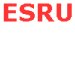Turbine Performance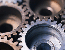Theory   Methodology   Conclusions   References

Theory

Performance of a turbine is characterised by the manner in which the three main indicators - power, torque and thrust - vary with tidal velocity. More emphasis is given to power in this analysis.

Total power output of the turbine depends on various parameters - Rotational Speed of the turbine (Ω), Tip Speed Ratio,(λ), Power Co efficient (Cp), Radius of the Turbine Blade(RT) and the tidal velocity.

The actual Power is calculated using the formula

P= 0.5 Cp ρ A V3

The tip speed ratio of the turbine is given by

λ= Ω RT / VT

Methodology

A computational analysis was carried out with an optimum blade design and a relation between the tip speed and Power Co efficient was obtained. This relation is used in further analysis.

In order to analyse the power output from the turbine we have adopted two main methods
1. Constant Rotational Speed Operation: When the rotational speed in maintained constant the tip speed ratio is also maintained constant. The power out put is strongly governed by the chosen operational speed. In this case the blades are fixed. If a low rotational speed is used the power reaches a maximum at lot tidal velocities and consequently it is very low.

2. Variable Speed Operation: If the speed of the rotor can be continuously adjusted such that the tip speed ratio remains constant at the level which gives maximum Cp the efficiency of the turbine will be significantly increased. Active pitch control is necessary to maintain a constant tip speed ratio thereby constant Cp but only in the process of adjustment of rotational speed. In order to attain high efficiency the pitch angle should always return to the optimum setting. An effective pitch control regulation is required in conditions above the rated speed when the rotational speed in kept constant. Such a turbine design would be expensive.
Through this analysis the value of such a turbine for use especially in sites with low and moderate tidal velocities was assessed.

Two locations were chosen for the analysis, one with high tidal velocities (Kyle of Rhea), and the other with low tidal velocities (Kintyre Peninsula South).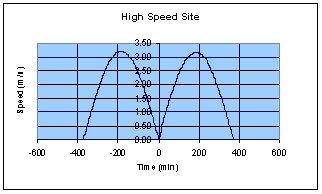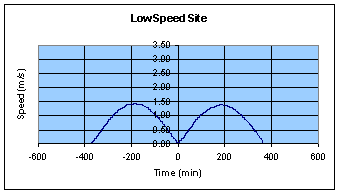With each of these locations total power output is calculated by running turbines at constant and variable speeds.

Conclusions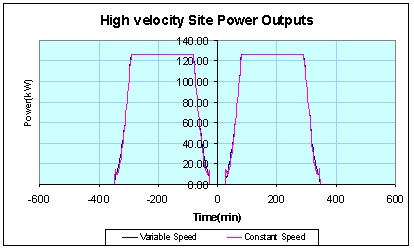The graph above shows the power out puts for the site with high tidal velocities. The turbine is run at a variable speed (constant Cp) and constant speed (variable Cp). In both the conditions the turbine reaches the rated speed.

 Energy Output (High Velocity Site) Value Units Annual Energy (Constant Speed ) 749.54 MWhr Annual Energy (Variable Speed) 754.09 MWhr Percentage Increase In Energy 0.61 %

The table above shows the difference in Annual Energy when turbine is run at Variable Speed and Constant Speed. When the velocity is high there is not much of difference whether the turbine is run at Variable Speed or at a Constant Speed.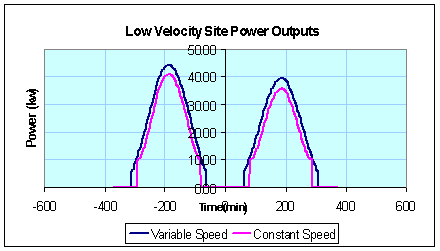The graph shows the power out puts for the site with low tidal velocities. When the turbine is run at a Variable Speed and a Constant Speed the rated speed is not reached. But there is considerable increase in power output.

 Energy Output (High Velocity Site) Value Units Annual Energy (Constant Speed ) 123.49 MWhr Annual Energy (Variable Speed) 151.49 MWhr Percentage Increase In Energy 22.67 %

In this table it is clearly seen that there is a considerable increase in annual energy when turbine is run at Variable Speed (constant Cp) than at Constant Speed (variable Cp). Therefore it can be observed that use of a turbine which runs at variable speed rather than at constant speed is justified when used in sites which have low tidal velocities.